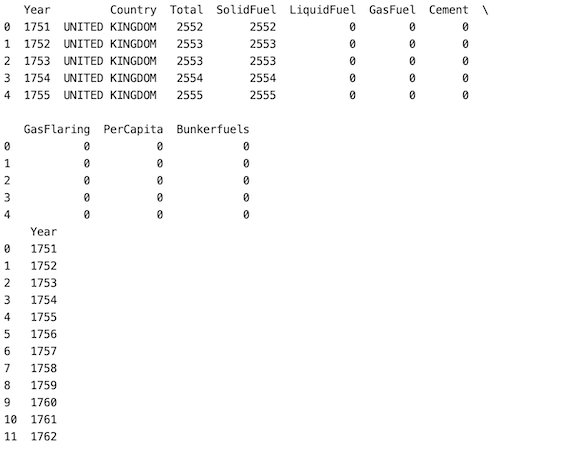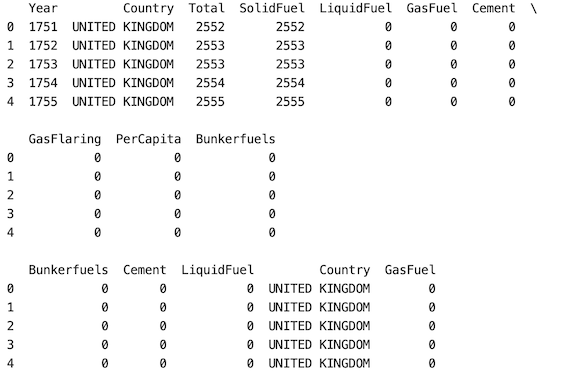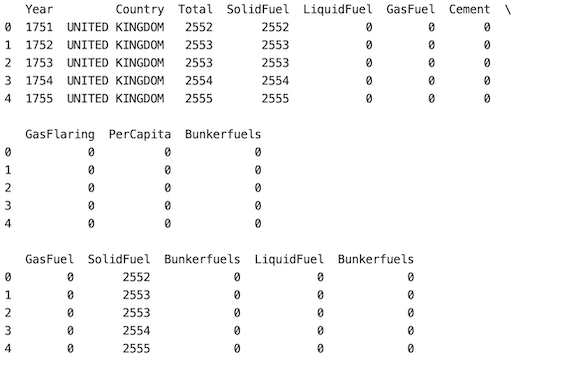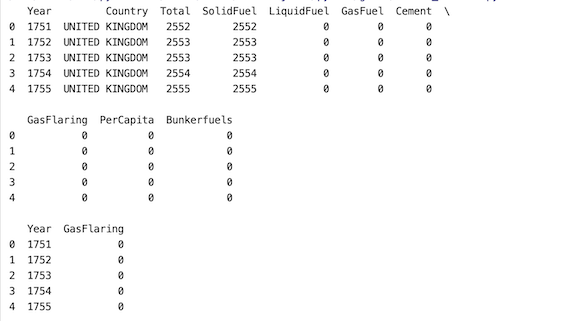Open in App
Not now

# Randomly Select Columns from Pandas DataFrame

• Last Updated : 28 Mar, 2022

In this article, we will discuss how to randomly select columns from the Pandas Dataframe.

According to our requirement, we can randomly select columns from a pandas Database method where pandas df.sample() method helps us randomly select rows and columns.

Syntax of pandas sample() method:

Return a random selection of elements from an object’s axis. For repeatability, you may use the random_state parameter.

DataFrame.sample(n=None, frac=None, replace=False, weights=None, random_state=None, axis=None)

Parameters:

• n: int value, Number of random rows to generate.
• frac: Float value, Returns (float value * length of data frame values ). frac cannot be used with n.
• replace: Boolean value, return sample with replacement if True.
• random_state: int value or numpy.random.RandomState, optional. if set to a particular integer, will return same rows as sample in every iteration.
• axis: 0 or ‘row’ for Rows and 1 or ‘column’ for Columns.

## Method 1: Select a single column at random

In this approach firstly the Pandas package is read with which the given CSV file is imported using pd.read_csv() method is used to read the dataset. df.sample() method is used to randomly select rows and columns. axis =’ columns’ says that we’re selecting columns. when “n” isn’t specified the method returns one random column by default.

## Python3

 `# import packages``import` `pandas as pd`` ` `# reading csv file``df ``=``pd.read_csv(``'fossilfuels.csv'``)``pd.set_option(``'display.max_columns'``, ``None``)``print``(df.head())`` ` `# randomly selecting columns``df ``=` `df.sample(axis``=``'columns'``)``print``(df)`

Output:## Method 2: Select a number of columns at a random state

In this approach, If the user wants to select a certain number of columns more than 1 we use the parameter ‘n’ for this purpose. In the below example, we give n as 5. randomly selecting 5 columns from the database.

## Python3

 `# import packages``import` `pandas as pd`` ` `# reading csv file``df ``=``pd.read_csv(``'fossilfuels.csv'``)``pd.set_option(``'display.max_columns'``, ``None``)``print``(df.head())``print``()`` ` `# randomly selecting columns``df ``=` `df.sample(n``=``5``, axis``=``'columns'``)``print``(df.head())`

Output:## Method 3: Allow a random selection of the same column more than once (by setting replace=True)

Here, in this approach, If the user wants to select a column more than once, or if repeatability is needed in our selection we should set the replace parameter to ‘True’ in the df.sample() method. Column ‘Bunkerfields’ is repeated twice.

## Python3

 `# import packages``import` `pandas as pd`` ` `# reading csv file``df ``=``pd.read_csv(``'fossilfuels.csv'``)``pd.set_option(``'display.max_columns'``, ``None``)``print``(df.head())``print``()`` ` `# randomly selecting columns``df ``=` `df.sample(n``=``5``, axis``=``'columns'``,replace``=``'True'``)``print``(df.head())`

Output:## Method 4: Select a portion of the total number of columns at random:

Here in this approach, if the user wants to select a portion of the dataset, the frac parameter should be used. In the below example our dataset has 10 columns. 0.25 of 10 is 2.5, it is further rounded to 2. A year and GasFlaring columns are returned.

## Python3

 `# import packages``import` `pandas as pd`` ` `# reading csv file``df ``=``pd.read_csv(``'fossilfuels.csv'``)``pd.set_option(``'display.max_columns'``, ``None``)``print``(df.head())``print``()`` ` `# randomly selecting columns``df ``=` `df.sample(frac``=``0.25``, axis``=``'columns'``)``print``(df.head())`

Output:My Personal Notes arrow_drop_up